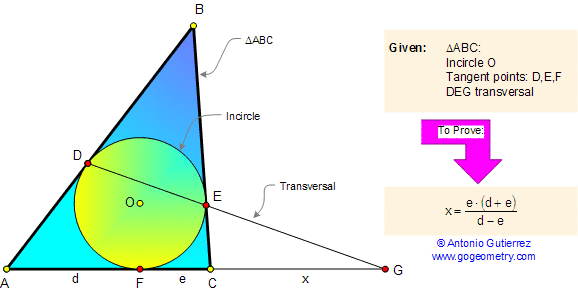# Geometry Problem 64: Triangle, Circle, Tangent, Transversal. High School, SAT Prep, College

< PREVIOUS PROBLEM  |  NEXT PROBLEM >

 In the figure below, let ABC be a triangle, the inscribed circle (incircle) is tangent to each side at D, E, and F. The line DE meet AC at G. If AF = d, FC = e and CG = x prove that:Home | Geometry | Search | Problems | 61-70 | Circle Tangent Line | Email | by Antonio Gutierrez